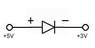# Voltage drop across diode

• NotStine

#### NotStine

Hi guys,

I have a very simple question but which is messing with my mind.

I'm trying to figure out the voltage drop across a diode when it is reverse biased, and I'm just not getting anywhere. Can someone please take a look at the attached image and explain to me what the voltage drop across the diode will be and why?

I just cannot get my head around this topic. Actually I'm not even sure if this diode will be reverse biased lol. That's the kind of level I'm currently at. If +5V goes from the anode side, that makes it forward biased right? But then there is +3V coming from the cathode side making it reverse biased?

Any help would be appreciated.

Thanks

#### Attachments

•Untitled-1.jpg
1.7 KB · Views: 1,912
Normal silicon diodes have 0.6 v drop. Some of the blue or UV LED have 4.5 v drop.

where is this diode used?

this diode is not hooked up in reverse. The 5 volt side is more positive than the 3 volt side, and the diode is orientated in a forward biased fashion (current can flow through it) if that's how you want to look at it.

If the diode was in backwards, ideally no current would flow through it, so it would be open, and the there would be no voltage drop. It would be like measuring before and after the diode with nothing being there. It looks like this diode is in a circuit with something else as well, and the voltage drop is 2 volts. So I am guessing that the diode has a 2 volt drop, or break over point.

So if the anode side was less positive (e.g. +3V) than the cathode side (e.g. +5V), the diode would not conduct?

I took the above diode from the AND gate circuit below. I cannot figure out how this circuit works. I'm going to run through what I understand of it, and if somebody can please correct me if my analysis is wrong.

+5V goes goes to A,B and C (in green) because they are all parallel branchs and the same voltage is present at the top of parallel branchs. All of the diodes will conduct because +5V is more positive than +3V, +2V and +1V. The voltage drop across each diode will be 0.6V. So how is it that this circuit works? If all diodes conduct, isn't V = 0?

The correct answer is V = 1V, I = 4mA.

Some insight into this issue will be greately appreciated. Thanks.

#### Attachments

The ideal diode equation is

I = I0 exp[eV/kT]

where I = forward output current
e =electron charge
k = Boltzmann's constant
T = temperature in kelvin
V = forward voltage

so

V = (kT/e) Ln(I/I0)

where kT/e = 26 mV at room temp.

The reverse current is I0

So if the anode side was less positive (e.g. +3V) than the cathode side (e.g. +5V), the diode would not conduct?

I took the above diode from the AND gate circuit below. I cannot figure out how this circuit works. I'm going to run through what I understand of it, and if somebody can please correct me if my analysis is wrong.

+5V goes goes to A,B and C (in green) because they are all parallel branchs and the same voltage is present at the top of parallel branchs. All of the diodes will conduct because +5V is more positive than +3V, +2V and +1V. The voltage drop across each diode will be 0.6V. So how is it that this circuit works? If all diodes conduct, isn't V = 0?

The correct answer is V = 1V, I = 4mA.

Some insight into this issue will be greately appreciated. Thanks.

The diode connected to "1V" will cause the voltage at A, B, and C to be at about 1.6 to 1.7 V. So, the diodes connected to 2V and 3V will be reverse-biased and not conduct appreciably.

EDIT: reading your post more carefully, it looks like they take diode the voltage drop to be zero when forward-conducting. So while V will be 1.6 to 1.7 V in reality, it would be 1V in this idealized scenario.

The reason the first diagram is confusing is that such a situation cannot exist.
If a diode had 2 volts across it in the forward direction, it will normally fail (or has already failed).

Diodes with a series resistor to limit the current will normally have about 0.6 Volt across them (for silicon diodes).
If the source of voltage is less than this for silicon diodes or if it is negative and below the breakdown voltage of the diode, the current flow is essentially zero.
Power diodes conducting very large currents will sometimes have diode voltage drops of up to 1 volt.